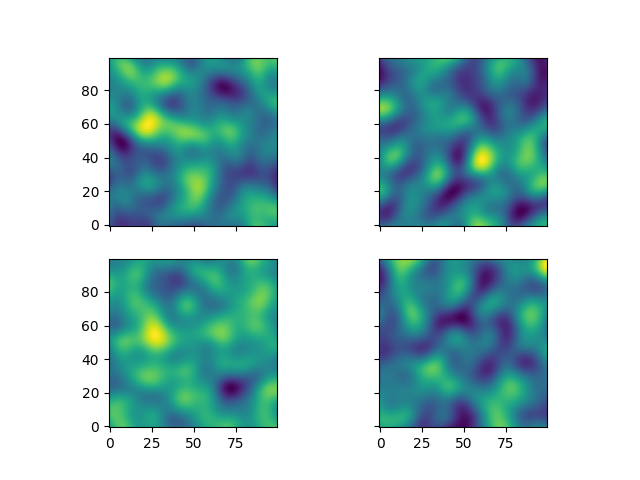# Creating an Ensemble of Fields¶

Creating an ensemble of random fields would also be a great idea. Let’s reuse most of the previous code.

```import numpy as np
import matplotlib.pyplot as pt
import gstools as gs

x = y = np.arange(100)

model = gs.Gaussian(dim=2, var=1, len_scale=10)
srf = gs.SRF(model)
```

This time, we did not provide a seed to `SRF`, as the seeds will used during the actual computation of the fields. We will create four ensemble members, for better visualisation and save them in a list and in a first step, we will be using the loop counter as the seeds.

```ens_no = 4
field = []
for i in range(ens_no):
field.append(srf.structured([x, y], seed=i))
```

Now let’s have a look at the results:

```fig, ax = pt.subplots(2, 2, sharex=True, sharey=True)
ax = ax.flatten()
for i in range(ens_no):
ax[i].imshow(field[i].T, origin="lower")
pt.show()
```## Using better Seeds¶

It is not always a good idea to use incrementing seeds. Therefore GSTools provides a seed generator `MasterRNG`. The loop, in which the fields are generated would then look like

```from gstools.random import MasterRNG

seed = MasterRNG(20170519)
for i in range(ens_no):
field.append(srf.structured([x, y], seed=seed()))
```

Total running time of the script: ( 0 minutes 4.932 seconds)

Gallery generated by Sphinx-Gallery